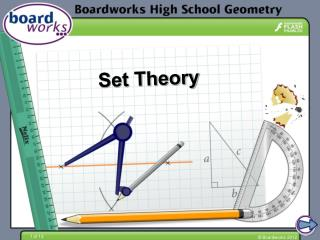# Set Theory - PowerPoint PPT PresentationDownload PresentationSet Theory

Set TheoryDownload Presentation## Set Theory

- - - - - - - - - - - - - - - - - - - - - - - - - - - E N D - - - - - - - - - - - - - - - - - - - - - - - - - - -
##### Presentation Transcript

1. Set Theory

2. Information

3. Sets and elements A set consists of objects or elements. The elements can be numbers, letters, etc. Elements are listed inside curly brackets. Sets can have a finite or infinite number of elements, or they can be empty. Empty sets, called the null set, have no elements and are denoted by the symbol ∅. A = {1, 2, 3, 4, 5, 6, 7, 8, 9} is a set of single digit positive numbers. B = {red, yellow, blue} is the set of primary colors C = {x|0 < x < 5} is the set of numbers between 0 and 5

4. Subsets What is the set of possible outcomes from rolling a die? A = {1, 2, 3, 4, 5, 6} What is the set of possible even outcomes? Ae = {2, 4, 6} The universal set is the largest possible set for a given scenario. For this example, Ais the universal set. Ae is a subset of all the possible outcomes, A, because is contains some of the elements of A. The null set is a subset of every set. Ae⊆A Subsets are denoted using the ⊆ symbol.

5. Complement The complement of a set is all of the elements in the universal set but not in the set of interest. Complements of sets are denoted using a prime symbol ′. What is the complement of the set of even outcomes when rolling a die? A = {1, 2, 3, 4, 5, 6} universal set: set of even outcomes: Ae = {2, 4, 6} complement of set: Ae′ = {1, 3, 5}, the set of possible odd numbers.

6. Unions The union of two or more sets contains all the elements in all the sets. The union of sets A and B is the elements in either set Aor B or both. Unions of sets are denoted by the symbol ∪. What is the union of set A, the outcomes of rolling a dice, and set B, all positive integers between 7 and 11? set A = {1, 2, 3, 4, 5, 6} set B = {7, 8, 9, 10, 11} A ∪ B = {1, 2, 3, 4, 5, 6, 7, 8, 9, 10, 11}

7. Intersections The intersection of two or more sets contains all the elements that are in all sets. For example, all the elements in set Aand B. Intersections of sets are denoted by the symbol ∩. What is the intersection of set A, the outcomes of rolling a dice, and set B, all positive integers between and inclusive of 4 and 9? set A = {1, 2, 3, 4, 5, 6} set B = {4, 5, 6, 7, 8, 9} A ∩B = {4, 5, 6,}

8. Disjoint sets Disjoint sets are two or more sets that have no elements in common, therefore the intersection is an empty set. For example: a set of all 3D shapes with a curved surfaces, and B, a set of all polyhedrons, are disjoint sets. The intersection of disjoints sets is the empty set: A∩B = ∅

9. Language of sets

10. Venn diagram

11. Unions and intersections Let A be the set of all letters in the alphabet. A = {A, B, C, …} Let B be the set of all vowels in the alphabet. B = { A, E, I, O, U} LetC be the set of all letters in your name. Draw a Venn diagram to represent these sets. What is the universal set and which are subsets? A is the universal set in this scenario. B is a subset of A. C is a subset of A. Describe B′. B′ is the set of all consonants in the English alphabet. List the elements in B ∩CandB ∪C?

12. Multiple sets

13. Sets in the animal kingdom